﻿ A New CFA Based Current-Mode Universal Biquad Filter

### A New CFA Based Current-Mode Universal Biquad Filter

Syed Naseem Ahmad, Anisur Rehman NasirOPEN ACCESSPEER-REVIEWED

## A New CFA Based Current-Mode Universal Biquad Filter

Syed Naseem Ahmad1, Anisur Rehman Nasir1,1Department of Electronics and Communication Engineering, Jamia Millia Islamia, New Delhi, India

### Abstract

The paper presents a new current-mode second order universal filter with single input and three outputs using two current feedback amplifiers, two grounded capacitors, two grounded resistors and only one floating resistor. The circuit implements lowpass, band-pass and high-pass filter functions simultaneously without changing configuration and passive components. All the passive components are grounded except one floating resistor. The grounded capacitors make circuit suitable for integration. The circuit has low active and passive sensitivities. Simulation results agree well with the theoretical analysis.

### At a glance: Figures

12
Prev Next

• Ahmad, Syed Naseem, and Anisur Rehman Nasir. "A New CFA Based Current-Mode Universal Biquad Filter." American Journal of Electrical and Electronic Engineering 2.2 (2014): 48-50.
• Ahmad, S. N. , & Nasir, A. R. (2014). A New CFA Based Current-Mode Universal Biquad Filter. American Journal of Electrical and Electronic Engineering, 2(2), 48-50.
• Ahmad, Syed Naseem, and Anisur Rehman Nasir. "A New CFA Based Current-Mode Universal Biquad Filter." American Journal of Electrical and Electronic Engineering 2, no. 2 (2014): 48-50.

 Import into BibTeX Import into EndNote Import into RefMan Import into RefWorks

### 1. Introduction

Analog filters find applications in many areas like communication, measurement, instrumentation and control system engineering. A current feedback amplifier(CFA)  is an active building block used to implementvoltageas well as current-mode analog signal processing circuits[1-10]. The low input impedance and high output impedance of CFA enables easy and simple realization of current-mode circuits. Some of the main advantages of current-mode circuits are small voltage swings at the nodes, excellent high frequency performance and larger dynamic range compared to voltage mode circuits. An added advantage is that filter current responses can be added or subtracted directly without any increase in the hardware. It is very suitable for portable equipment and gadgets where low value of supply voltage is required. The need to propose this new current feedback amplifier (CFA) based filter stems from the fact that single CFA multifunction filter proposed by Horng et al  suffers from low Q-values. In the proposed circuit two CFAs are used with only five passive elements out of which only one is floating. The quality factor is conveniently improved by the addition of an active element (CFA) in this circuit. Many other prominent current/voltage mode circuits employing CFAs are given in the literature [2-10].

In this paper, a new circuit configuration is proposed to realize current-mode second order lowpass, bandpass and highpass filters simultaneously. The notch and allpass filter functions are also obtained. The proposed filter circuit employs two CFAs, two grounded capacitors, two grounded resistors and one floating resistor. The proposed circuit has better quality factor in comparison with the Horng’s circuit . The active and passive sensitivities are low.

### 2. Proposed Circuit

The circuit symbol of CFA has been shown in Figure 1. The port relations of a CFA can be characterised by the following equations(1)

The proposed current-mode second order universal filter is shown in Figure 2. The routine analysis of the circuit using port relations of CFA yields the following current-mode transfer functions.(2)(3)(4)

Selecting the admittances as Y1=sC1, Y2=G2, Y3=G3, Y4=G4, and Y5=sC5, then the current transfer functions become(5)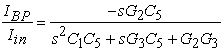(6)(7)

The circuit realizes highpass filter at IHP, a bandpass filter at IBP, and a lowpass filter at ILP simultaneously. The natural frequency ωO and quality factor Q are given by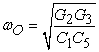(8)(9)

From eqn. (8) and eqn. (9) it is evident that the natural angular frequency ωO and quality factor Q of the current-mode filter are dependent on each other, that is whenever ωO is changed, the value of quality factor Q gets disturbed. But if ratio of either C1 and C5 or G2 and G3 is kept constant, then ωO can be varied without disturbing the quality factor. The direct addition of IHP and ILP yields current-mode notch filter where as allpass filter can be obtained directly from the three available responses i.e. IHP, ILP and IBP without any additional hardware.

### 3. Non-ideal Equivalent Circuit of CFA

Figure 3 shows the non-ideal equivalent circuit model of the CFA. Rx is the input resistance of the x-terminal, Ry// (1/sCy) represents the y-terminal parasitic input impedance and Rp// (1/sCp) gives the parasitic impedance value at z-terminal . The values of the various parasitics for the bipolar CFAs (AD844) are Rx=50Ω, Cp=5.5pF, Rp= 3MΩ, Ry=2MΩ and Cy=2pF. The effect of these parasitic impedences is prominent at sufficiently high frequencies.

### 4. Sensitivity Analysis

Taking into account the tracking errors of current feedback amplifier, the terminal equation (1) get modified as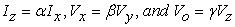(10)

wheredenotes the current tracking error,is the input voltage tracking error andis the output voltage tracking error of the current feedback amplifier. The natural angular frequency ωO, quality factor Q and bandwidth of filter of Figure 2 becomes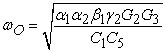(11)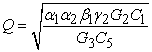(12)(13)

The active and passive sensitivities of the proposed filter have been found to be fairly small and are computed below.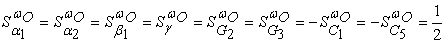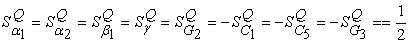From above results it is obvious that the active and passive sensitivities of the proposed filter are low and not larger than unity.

### 5. Simulation Results

The proposed current-mode second order universal biquadratic filter has been simulated with TINA Pro simulation program. The universal filter has been designed for fO = 10.5KHz and Q=1. The CFA has been realized with commercially available AD844s. The values of passive components used are C1=C5=10nF, R2=R3= R4=1kΩ, The supply voltages are ±12V.The simulated magnitude responses of lowpass, bandpass, highpass and notch filters are shown in Figure 4. The phase response of second order allpass filter is shown in Figure 5. The simulation results agree well with theoretical analysis of the filter.

### 6. Conclusions

The aim of realizing proposed circuit has been to improve the single CFA filter given by Horng et al  where single CFA is used with six passive elements out of which two resistors are floating. The quality factor of Horng et al  is poor and remains less than half for any combination of passive component values. Secondly number of passive elements in this circuit is large and also number of floating resistors are two which is a limitation of using single CFA. However in the proposed circuit as the number of CFAs used are increased to two the number of passive elements is reduced to five and also number of floating components is reduced to only one. On the other hand quality factor is also improved in the proposed circuit and can be set by either G2 or by ratio of C1/C5. Other major achievement is that all the filter responses can be obtained without any extra hardware which can be seen in the results of Figure 4.

As a whole the proposed circuit is a compact circuit providing all the current-mode filter responses with minimum number of floating passive elements. The novel idea here is that the input is indirectly applied to low impedance node of CFA through RC network. The active and passive sensitivities of the proposed filter are low and less than unity. Simulation results confirm the theoretical analysis.

### References

  C. Toumazou, F. J. Lidgey and D. G. Haigh (ed.),“Analogue IC Design: The current mode Approach”, London, Peter Peregrinus Ltd, 1990.In article  J.W. Horng, P.Y. Chou and J.Y. Wu, “Voltage/current-mode multifunction filters using current feedback amplifiers and grounded capacitors”, Active and Passive Electronic components, vol.2010, p.1-5, 2010.In article CrossRef  K. Kumar and K.Pal, “High input impedance voltage mode universal biquad filter,” Indian Journal of Pure and Applied Physics, vol.48, no.4, p.292-296, 2010.In article  J. W. Horng, C.L Hou, W. S. Huang, and D. Yang, “Voltage/Current-Mode Multifunction Filters Using One Current Feedback Amplifier and Grounded Capacitors”, Circuits and Systems, vol.2, no.2, p.60-64, April 2011.In article CrossRef  A. Fabre, “Insensitive voltage-mode and current-mode ﬁlters from commercially available trans-impedance op amps,” IEE Proceedings G, vol. 140, p. 319-321, Oct. 1993.In article  S.I. Liu, “High input impedance ﬁlters with low component spread using current-feedback ampliﬁers.” Electronics Lett, vol. 31, no. 13, p.1042-1043, 1995.In article CrossRef  R. K. Sharma and R. Senani, “Universal current mode biquad using a single CFOA”, Int. J. Electronics, Vol. 91, No. 3, , 175–183, March 2004.In article CrossRef  S.S. Gupta, D.R.Bhaskar and R. Senani, “New Volyage controlled oscillators using CFOAs”, Int. J. Electron Commun AEU, 63,3 p.209-217, 2009.In article  S.N. Ahmad, M.R. Khan and Moinuddin, “A new current-mode universal filter , Frequenz, vol.7/8,195-196,2004In article  N.A. Shah, M.F. Rather and S.Z. Iqbal, “Current-mode universal filter using CFAs”, Indian J. Pure and Applied Physics, vo. 42, p.375-377, 2004.In article